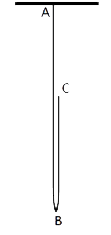Math helps to fight functional illiteracy.
47% of the Italians, as OCSE says, soffers of functional illiteracy and the percent is so high to make our country skip to the top of the world chart.

Mathematical skills have been recognised as foundamental to the personal fulfilment, the ative citizenship, the social inclusion and the employability in the society of knowledge.
(Official Gazette of the EU, L 394 series, 12.30.2006)

Science teaches us not to neglect anything, not to scorn the modest beginnings, because in little things are always present the principles of the big ones, as in the big is always contained the little.
(Michael Faraday, English Physician and Chemist, 1791-1867)

## much more

ideas, curiosities, deepenings.

### Scientific articlesTHE SQUARE OF A SECOND-DEGREE TRINOMIAL (Teacher Valentina Fabbro):

The article presents a standard to establish if a fourth-degree polynomial which coefficients are Real numbers is the development of a second-degree polynomial which coefficients are Real numbers; if it is so, there is a general formula to determine the second-degree polynomial starting from the coefficients of the original fourth-degree polynomial.

CONGRUENT TRIANGLES (Teacher Valentina Fabbro):

The article presents a demonstration of the following statement: two triangles are congruent if they have congruent two sides and the bisector of the angle included. The need of this demonstration was born from a quiz, found in a Geometry book that contained a mistake.

THE TANGRAM OF THE POLYGONS (Gioella Lorenzon and Teacher Fabio Breda):

The aim of this article is to produce a general method that allows, given to whatsoever equivalent polygons, to equitable break them. In order to do this we will demonstrate Bolyai's theorem that is that equivalent polygons are equitable breakable, and we will then conclude that for what pertains the polygons' equivalence and equidecomposability are the same relations.

### CuriositiesFrom Pythagoras to Gödel the recurrent question about numbers is always the same: did they already exist before we discovered them or are they just an invention? (by Carlo Rovelli)

Does a famous scientist share my birth date? Will I follow his career? (by Teacher Fabio Breda)

Does a famous scientist share my birth date? Will I follow his career? (by Teacher Fabio Breda)

### Problem of the monthPROBLEM DECEMBER 2014:

Find how many couples of integers satisfie at the same time these two conditions:

• the sum of their squares is minor than 13;

• the sum of their absolute values is major or equal to 4.

• Can we change the number 13 in order to have exactly four couples of integers?

PROBLEM SEPTEMBER 2014:

 A rope which length is l meters is bonded to the ceiling trough one of its endings. The other ending is pulled up vertically in a way for the rope to be disposed as in the figure. If the free ending is pulled up of n meters, how much does the lowest point of the rope ascend? Suppose that segments AB and BC, whose sum is obviously l, are perfectly parallel.PROBLEM MARCH 2014:

Rewrite the following polynomials as squares:

• x4 + 6x3 + 17x2 + 24x + 16;

• x4- 8x3 + 26x2 - 40x + 24.

The students that want to try to solve the problem of the month, will have to deliver their proposal, the way they prefer with a wide and justified motivation, to the teachers who run the ArchimedeProject. The correct and better-argued answer will be published in this section of the site. Have fun!!